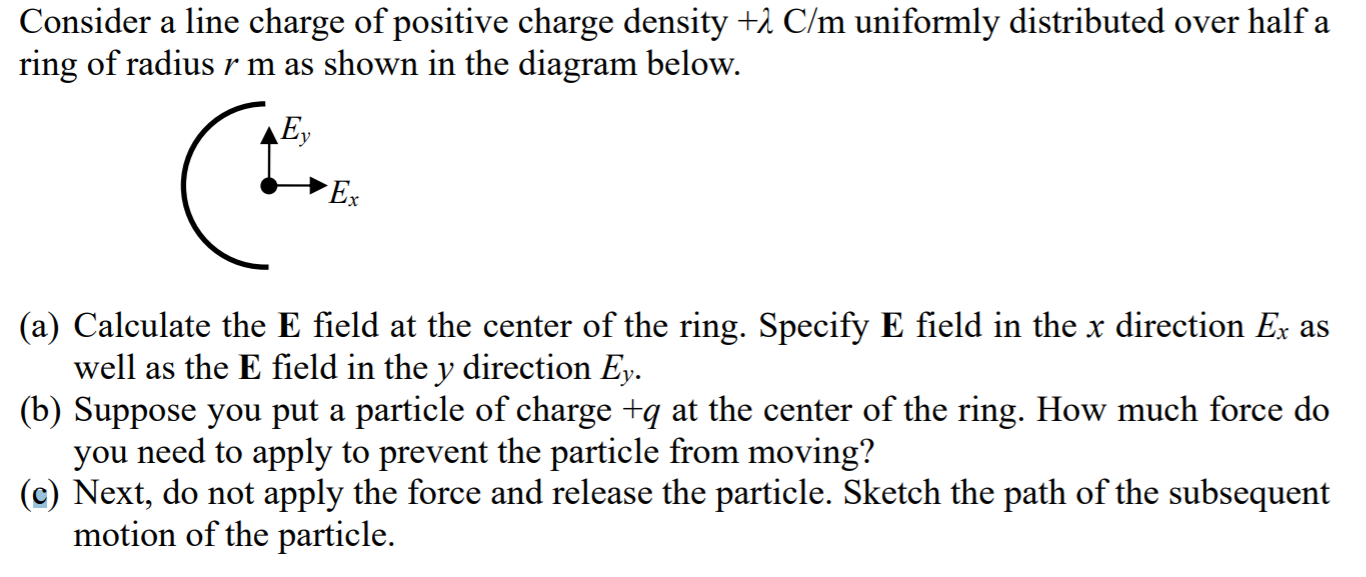Home / Answered Questions / Other / consider-a-line-charge-of-positive-charge-density-h-c-m-uniformly-distributed-over-half-a-ring-of-ra-aw769

# (Solved): Consider A Line Charge Of Positive Charge Density +h C/m Uniformly Distributed Over Half A Ring Of R...Consider a line charge of positive charge density +h C/m uniformly distributed over half a ring of radius r m as shown in the diagram below. (a) Calculate the E field at the center of the ring. Specify E field in the x direction Ex as well as the E field in the y direction Ey. (b) Suppose you put a particle of charge +q at the center of the ring. How much force do you need to apply to prevent the particle from moving? (c) Next, do not apply the force and release the particle. Sketch the path of the subsequent motion of the particle.

We have an Answer from Expert